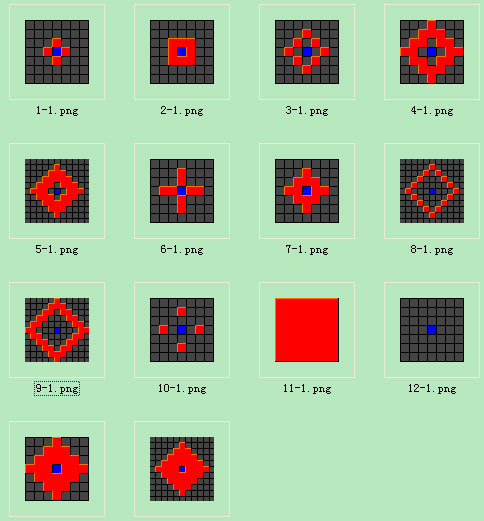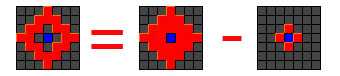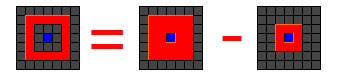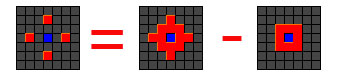……

/// <summary>

/// 战斗范围类型

/// </summary>
public enum AttackRangeTypes {

/// <summary>

/// 无

/// </summary>
None = 0,

/// <summary>

/// 全八面(方形)

/// </summary>
Square = 1,

/// <summary>

/// 斜四面(菱形)

/// </summary>
Diamond = 2,

/// <summary>

/// 正四向(十字)

/// </summary>
Cross = 3,

/// <summary>

/// 斜四向(交叉)

/// </summary>
Oblique = 4,
}

None外，它们2长度范围分别对应以下图例：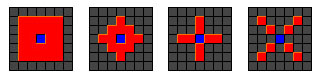List<Point> GetRange(Point center, AttackRangeTypes attackRangeType, int range) {
List
<Point> points = new List<Point>();

for (int x = -range; x <= range; x++) {

for (int y = -range; y <= range; y++) {

switch (attackRangeType) {

case AttackRangeTypes.None:

continue;

case AttackRangeTypes.Square:

break;

case AttackRangeTypes.Diamond:

if (Math.Abs(x) + Math.Abs(y) > range) { continue; }

break;

case AttackRangeTypes.Cross:

if (Math.Abs(x) != 0 && Math.Abs(y) != 0) { continue; }

break;

case AttackRangeTypes.Oblique:

if (Math.Abs(x) != Math.Abs(y)) { continue; }

break;
}
new Point(center.X + x, center.Y + y));
}
}

return points;
}

/// <summary>

/// 获取攻击范围坐标列表

/// </summary>

/// <param name="rangeType">范围类型</param>

/// <param name="range">范围</param>

/// <param name="exclusionRangeType">排除范围类型</param>

/// <param name="exclusionRange">排除范围</param>

/// <returns></returns>
public List<Point> AttackRange(AttackRangeTypes rangeType, int range, AttackRangeTypes exclusionRangeType, int exclusionRange) {
List
<Point> points = GetRange(Coordinate, rangeType, range);
List
<Point> excludePoints = GetRange(Coordinate, exclusionRangeType, exclusionRange);

return points.Except(excludePoints).ToList();
}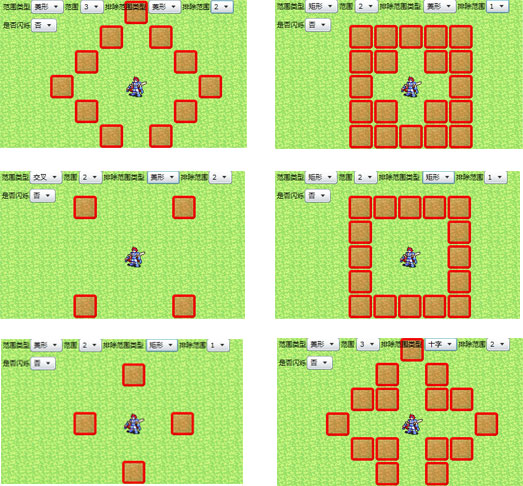……

posted on 2011-08-19 23:50  深蓝色右手  阅读(9378)  评论(24编辑  收藏  举报